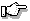# calloc(), _bcalloc(), _fcalloc(), _ncalloc()

allocate space for an array

## Synopsis:

```#include <stdlib.h>  /* For ANSI compatibility
(calloc only) */
#include <malloc.h>  /* Required for other
function prototypes */
void *calloc ( size_t n, size_t size );
void __based (void) *_bcalloc ( __segment seg,
size_t n,
size_t size );
void __far  *_fcalloc ( size_t n, size_t size );
void __near *_ncalloc ( size_t n, size_t size );```

## Description:

The calloc() functions allocate space for an array of n objects, each of length size bytes. Each element is initialized to 0.

Each function allocates memory from a particular heap, as listed below:

calloc()
Depends on data model of the program (see below)
_bcalloc()
Based heap specified by the value of seg
_fcalloc()
Far heap (outside the default data segment)
_ncalloc()
Near heap (inside the default data segment)

In a small data memory model, calloc() is equivalent to _ncalloc(); in a large data memory model, calloc() is equivalent to _fcalloc().A block of memory allocated with one of the calloc() functions should be freed using the corresponding free() function.

## Returns:

A pointer to the start of the allocated memory, or NULL (_NULLOFF for _bcalloc()) if there isn't sufficient memory available or if the value of the size argument is zero.

## Examples:

```#include <stdlib.h>

int main( void )
{
char *buffer;

buffer = (char *)calloc( 80, sizeof(char) );
return( EXIT_SUCCESS );
}```

## Classification:

calloc() is ANSI; _bcalloc(), _fcalloc() and _ncalloc() are WATCOM.
Safety:
Interrupt handler No
Signal handler No